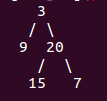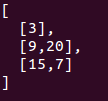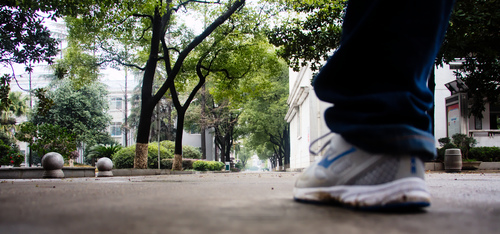I try to collect problems about `Binary Search Tree (BST)` which are asked in interview frequently.

Basicly, here is the definition of Binary Search Tree. And I show the way how to implement the basic operation like `insert`, `delete` and so on.

You could find all my practices with BST in github: BSTThere are three different ways to travel a BST. N(node), L(left subtree), R(right subtree)

• NLR: Firstly the traveller access the data of the Node(N) and then it enter into the left sub-tree, travel the right sub-tree

• LNR

• LRN

It’s very easy and obvious to implement the recursive definition of the three different traveller.

You will find that it’s a very efficient way to understand what means Pre-traveller, In-traveller and Post-traveller.

But the interview officer may not be satisfy with your recursive implementation. Take some time to understand the iteratly implementation below there.

There is another interesting problem that how to travel a BST in level order. Something like this:
For example:

Given binary tree {3,9,20,#,#,15,7},return its level order traversal as:At this moment, you should try to use some basic data structure to solve this problem. Don’t forget STACK :)

After you solve this problem, you have knew that stack is a efficient and useful ADT. Once you meet a hard problem, try to use another ADT to solve your problem.

Function `isValidBST` help us to check whether the tree is a BST. Now, if you have no idea about what means a BST, go to wikipedia and help yourself :)

You know that if we travel the tree with `In-Order traveller`, the output of the traveller is sorted from small element to big one.

There is a joke about how to invert a BST.

Google: 90% of our engineers use the software you wrote (Homebrew), but you can’t invert a binary tree on a whiteboard so fuck off.

It’s easy to solve this problem by recursion.

You may also be asked to translate a sorted array into BST. So, how to make it?

How to use the output of `InOrder-Traveller` and `PostOrder-Traveller` to rebuild a BST ?

Photo By Jason Leaster in ChangDe, China